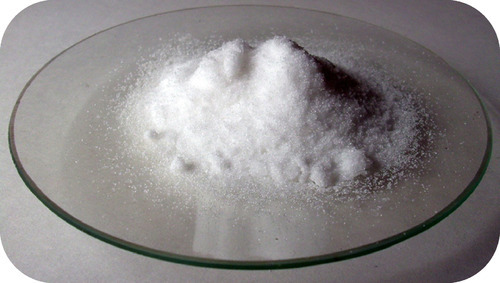## Dissociation

### Learning Objectives

• Define dissociation.
• Be able to write equations for dissociation of ionic compounds.

### Have you ever seen trucks pour salt on icy roads?Runner on a Frozen Road. Courtesy of FEMA.

In many areas, ice on the streets and sidewalks represent a serious walking and driving hazard.  One common approach to melting the ice is to put some form of deicing salt on the surface.  Materials such as sodium chloride or calcium chloride are frequently employed for this purpose.  In order to be effective, the solid material must first dissolve and break up into the ions that make up the compound.

## Dissociation

An ionic crystal lattice breaks apart when it is dissolved in water.  Dissociation is the separation of ions that occurs when a solid ionic compound dissolves.  It is important to be able to write dissociation equations.  Simply undo the crisscross method that you learned when writing chemical formulas of ionic compounds.  The subscripts for the ions in the chemical formulas become the coefficients of the respective ions on the product side of the equation.  Shown below are dissociation equations for NaCl, Ca(NO3)2 , and (NH4)3PO4.

NaCl(s) → Na+ (aq) + Cl (aq)

Ca(NO3) 2 (aq) → Ca2+ (aq) + 2NO3 (aq)

(NH4)3 PO4 (s) → 3NH4 + (aq) + PO4 3- (aq)

The formula unit of sodium chloride dissociates into one sodium ion and one chloride ion.  The calcium nitrate formula unit dissociates into one calcium ion and two nitrate ions.  This is because of the 2+ charge of the calcium ion.  Two nitrate ions, each with a 1− charge are required to make the equation balance electrically.  The ammonium phosphate formula unit dissociates into three ammonium ions and one phosphate ion.  Note that the polyatomic ions themselves do not dissociate further, but remain intact.

Do not confuse the subscripts of the atoms within the polyatomic ion for the subscripts that result from the crisscrossing of the charges that make the original compound neutral.  The 3 subscript of the nitrate ion and the 4 subscript of the ammonium ion are part of the polyatomic ion and simply remain as part of its formula after the compound dissociates.  Notice that the compounds are solids ( s ) which then become ions in aqueous solution ( aq ).Figure 1. Calcium nitrate is a typical ionic compound. In an aqueous solution it dissociates into calcium ions and nitrate ions. By Ondřej Mangl.

Nonelectrolytes do not dissociate when forming an aqueous solution.  An equation can still be written that simply shows the solid going into solution.  For the dissolving of sucrose:

C 12 H 22 O 11 (s) → C 12 H 22 O 11 (aq)

### Summary

• Dissociation is the separation of ions that occurs when a solid ionic compound dissolves.
• Nonionic compounds do not dissociate in water.

### Practice

http://www.chemistrylecturenotes.com/html/dissociation_of_ionic_compound.html

1. What does silver nitrate form upon dissociation in water?
2. How would HCl dissociate in water?
3. Is HF primarily dissociated or undissociated in water?
4. Do alcohols dissociate in water?

### Review

1. Define dissociation.
2. Do polyatomic ions dissociate when dissolved in water?
3. Write the equation for the dissociation of KBr in water.
4. Write the equation for the dissociation of NH 4 Cl in water.

## Glossary

• dissociation:  The separation of ions that occurs when a solid ionic compound dissolves.